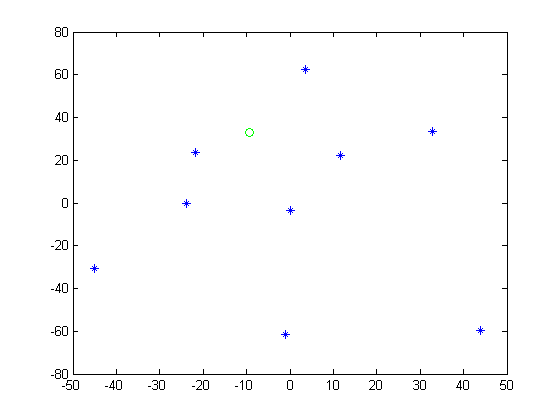# brief theory

Earthquake hypocenter location is an important task in seismology. In the past seismologists had to use geometrical methods (e.g. Circle methods) to find it. It wasn't until the proposal of inversion theory that a new perspective of solving this problem was implemented. The core of this technique assumes that given a set of data we can obtain the model that those data represents based on

d=Gm

Where d is a matrix containing the data with size(nx1)

G is a matrix containing the change in the model with size (nxm)

m is the model matrix with size(mx1)

If G is square, which implies we have a system of n=m equations and n=m unknowns, we could simply multiply both sides of the equation by G -1 the inverse of G
So we would have
G -1 d=m

However, this is not the case in seismology where the number of unknowns is huge. Therefore the matrices are not squares.So, this problem has to be solve by using inversion techniques.Fortunately, nowadays there are several tools available too perform inversions. Here we shall focus on how to find an eathquake hypocenter using matlab.

## Data

Let's say there are 10 stations to which data(seismograms), you have access

Here is the data for station coordinates and arrival times arrival_times.txt

Column 1: Station name
Column 2: longitude (km)
Column 3: latitude (km)
Column 4: height (m) at station site
Column 5: P wave arrival time (s)
Column 6: S wave arrival time (s)
Column 7: S-P wave arrival time (s)
Column 8: P wave velocity (km/s)
Column 8: S wave velocity (km/s)

Let’s import these files in Matlab.
%assigning values
A = importdata(
'arrival_times.txt', '\t', 1);
tp=A.data(:,4);
ts=A.data(:,5);
v2=[A.data(:,7);A.data(:,8)];
name = A.textdata(:,1);
xco = [A.data(:,1);A.data(:,1)];
yco = [A.data(:,2);A.data(:,2)];
zco = [A.data(:,3)/1000;A.data(:,3)/1000];

Declare some variables
%Definition of data vector matrix, Kernel matrix, and others
G=zeros(length(xco),4);
dobs=zeros(length(xco),1);
dtdx=zeros(length(xco),1);
dtdy=zeros(length(xco),1);
dtdz=zeros(length(xco),1);
dtdt=zeros(length(xco),1);
arrvt=zeros(2*length(xco),1);
dpred=zeros(length(xco),1);
d=zeros(length(xco),1);
res=zeros(length(xco),1);
mic=zeros(100000,4);
dmn=zeros(4,1);
mestn=zeros(4,1);
OT=187.2;
xg=val(1,1);
yg=val(1,2);
zg=10;
tg=OT;
mi=[xg;yg;zg;tg];
cont=0;
arrvt=[tp;ts];

xg, yg, and zg, corresponds to the intial guess which is a value that(for the first calculation) one have to propose, in this example this value is on the file val.txt

Let’s plot the 10 seismic stations.
% Plotting stations locations
figure(1);
plot(xco(:,1),yco(:,1),'b*')
hold onFigure 1. Seismic stations location.

Let’s plot our initial guess.
% Plotting the initial guess as a green circle
figure(2);
plot(xco(:,1),yco(:,1),'b*')
hold on
plot(xg,yg,'go')Figure 2. Green circle represents our initial guess for the earthquake hypocenter location.

Algorithm to find the solution

As mentioned before, To solve this problem we have to use the generalized inverse theory, so we need to construct three matrices
First of all the data matrix
d=[t1,t2,t3..tn] where t1,t2,t3...tn are arrival times in station 1, station 2 and so on respectively.
then the kernel matrix
G=[dti/dx,dti/dy,dti/dz,dti/dt] where dti/dx,dti/dy,dti/dz,dti/dt are partial derivatives with respect to time.
Finally the model matrix
m=[mx,my,mz,mt] where mx,my,mz,mt are for the first iteration the initial guess values this is
mx=xg
my=yg
mz=zg
mt=tg

and after the first iteration the model matrix must be updated with the inverted one each time.

Let’s see the code
% definition of the intial minimum error
E=10;
%begin the iterations
while min(abs(E))>(1*10^0);
cont=cont+1;
if cont<=1
dobs(:)=[tp(:);ts(:)];
for i=1:18
alpha=v2(i);
% calculating the partial derivatives
dtdx(i)=(-(xco(i)-mi(1)));
dtdx(i)=dtdx(i)/(alpha^2*(arrvt(i)-mi(4)));
dtdy(i)=(-(yco(i)-mi(2)));
dtdy(i)=dtdy(i)/(alpha^2*(arrvt(i)-mi(4)));
dtdz(i)=(-(zco(i)-mi(3)));
dtdz(i)=dtdz(i)/(alpha^2*(arrvt(i)-mi(4)));
dtdt(i)=1;
% travel time calculation
ttt(i)=sqrt(((xco(i)-xg)*(xco(i)-xg))+((yco(i)-yg)*(yco(i)-yg))+((zco(i)-zg)*(zco(i)-zg)))/alpha;
dpred(i)=OT+ttt(i);
d(i)=dobs(i)-dpred(i);
end
G=[dtdx dtdy dtdz dtdt];
% Getting Eigenvalues and Eigenvectors of G
[U,S,V] = svd(G);
% Least square solution
dm=inv(G'*G)*G'*d;
% updating the 'mi' vector
mi=mi+dm;
mic(cont,:)=(mi(:))';
dc=G*mi;
E=(sum(d(:)).^2);
% Plot first solution as a cyan circle
figure(3);
plot(xco(:,1),yco(:,1),
'b*')
hold on
plot(xg,yg,'go')
hold on
plot(mi(1),mi(2),
'co')

After the first iteration (cyan circle) you can notice how the earthquke position has changed in comparison to our intial guess (green circle)Figure 3. Earthquake hypocenter after the first iteration.

As surely you have noticed the new model matrix has been changed, this assure the next steps in the iteration will have a new "guessed model", but this time it is originated from the inversion

Now, lets look at the second part of the solution, which is pretty much the same as the first part

% Creating a new figure for the next stage
% and plotting first solution as a cyan circle

figure(4);
plot3(mi(1),mi(2),-mi(3),
'co')
hold
on
% evalauting condition before starting next iterations
elseif cont>1
dobs(:)=[tp(:);ts(:)];
for i=1:18
alpha=v2(i);
% calculating the partial derivatives
dtdx(i)=(-(xco(i)-mi(1,1)));
dtdx(i)=dtdx(i)/(alpha^2*(arrvt(i)-mi(4)));
dtdy(i)=(-(yco(i)-mi(2,1)));
dtdy(i)=dtdy(i)/(alpha^2*(arrvt(i)-mi(4)));
dtdz(i)=(-(zco(i)-mi(3,1)));
dtdz(i)=dtdz(i)/(alpha^2*(arrvt(i)-mi(4)));
dtdt(i)=1;
% travel time calculation
ttt(i)=sqrt(((xco(i)-mi(1,1))*(xco(i)-mi(1,1))+((yco(i)-mi(2,1))*(yco(i)-mi(2,1)))+((zco(i)-mi(3,1))*(zco(i)-mi(3,1))))/alpha;
dpred(i)=mi(4)+ttt(i);
d(i)=dobs(i)-dpred(i);
end
G=[dtdx dtdy dtdz dtdt];
% Getting Eigenvalues and Eigenvectors of G
[U,S,V] = svd(G);
% Least square solution
dm=inv(G'*G)*G'*d;
% updating the 'mi' vector
mi=mi+dm;
mic(cont,:)=(mi(:))';
dc=G*mi;
E=(sum(d(:)).^2);
end
end
%deleting zeros rows in the mic matrix
av=mic;
av(~any(mic,2),:)=[];
mic=av;
clear
av

Then,all we need is just to follow small details for graphing(a work around to get different colours in the same graph)
for i=2:cont-1
% Plot each iteratively calculated solution as a blue circle
plot3(mic(i,1),mic(i,2),-mic(i,3),
'o','Color',[0 0 1]);
end

In order to know how many iterations took the routine to get over the minimum error constrain

sprintf(
' # of iterations %d',cont)

Finally, plot the best solution as a red circle

hold on
plot3(mic(cont,1),mic(cont,2),-mic(cont,3),
'o','Color',[1 0 0]);
xlabel(
'X'),ylabel('Y'), grid;
hold on
plot3(xco(:,:),yco(:,:),-zco(:,:),
'*','Color',[0 0 1])

The final graph should look like```Figure 4. Earthquake hypocenter from inversion(Red circle).Note the change in depth after each iteration(blue circles, Fig. 5). This has been a clear example of how to use computational tools for solving real life problems.Here is the script for this example hypocenter.txt after saving the file, just change the".txt" extension to ".m" and it will work fine. Finally, a graphical review of the calculations is shown belowFigure 5. Earthquake hypocenter from inversion.Note the change in depth after each iteration.Red circle represents the best solution.    Please post comments about this page here
```